# imresize

## Syntax

``B = imresize(A,scale)``
``````B = imresize(A,[numrows numcols])``````
``````[Y,newmap] = imresize(X,map,___)``````
``___ = imresize(___,method)``
``___ = imresize(___,Name,Value)``

## Description

example

````B = imresize(A,scale)` returns image `B` that is `scale` times the size of `A`. The input image `A` can be a grayscale, RGB, or binary image. If `A` has more than two dimensions, `imresize` only resizes the first two dimensions. If `scale` is in the range [0, 1], `B` is smaller than `A`. If `scale` is greater than 1, `B` is larger than `A`. By default, `imresize` uses bicubic interpolation.```

example

``````B = imresize(A,[numrows numcols])``` returns image `B` that has the number of rows and columns specified by the two-element vector `[numrows numcols]`. ```

example

``````[Y,newmap] = imresize(X,map,___)``` resizes the indexed image `X` where `map` is the colormap associated with the image. By default, `imresize` returns a new, optimized colormap (`newmap`) with the resized image. To return a colormap that is the same as the original colormap, use the `'Colormap'` parameter. ```

example

````___ = imresize(___,method)` specifies the interpolation method used. ```
````___ = imresize(___,Name,Value)` returns the resized image where `Name,Value` pairs control various aspects of the resizing operation.```

## Examples

collapse all

`I = imread('ngc6543a.jpg');`

Shrink the image by a factor of two.

`J = imresize(I, 0.5);`

Display the original image and the resized image.

`figure, imshow(I), figure, imshow(J)`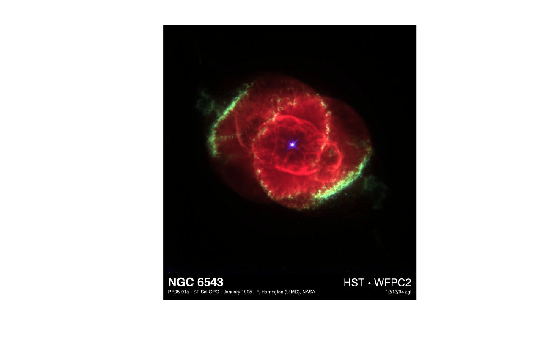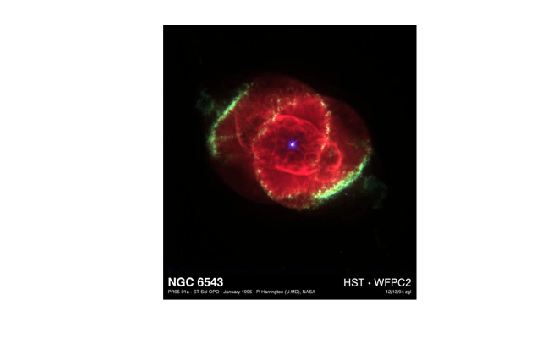Load an image into the workspace.

`I = imread('ngc6543a.jpg');`

Shrink by factor of two using nearest-neighbor interpolation. This is the fastest method, but it has the lowest quality.

`J = imresize(I, 0.5, 'nearest');`

Display the original image and the resized image.

```figure imshow(I)``````figure imshow(J)```Read an RGB image into the workspace.

`RGB = imread('peppers.png');`

Resize the RGB image to have 64 rows. `imresize` calculates the number of columns automatically.

`RGB2 = imresize(RGB, [64 NaN]);`

Display the original image and the resized image.

```figure imshow(RGB)```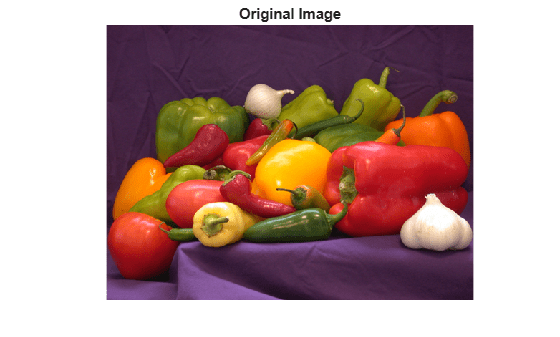```figure imshow(RGB2)```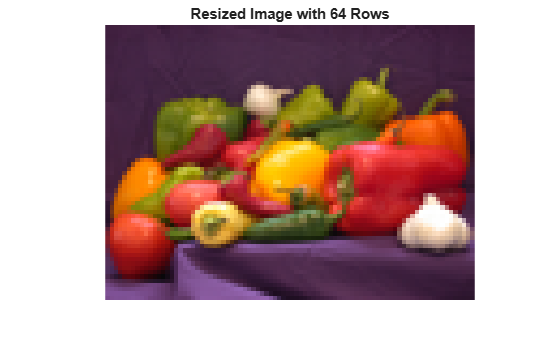Read an indexed image into the workspace.

`[X, map] = imread('corn.tif');`

Shrink the indexed image by a factor of two.

`[Y, newmap] = imresize(X, map, 0.5);`

Display the original image and the resized image.

```figure imshow(X,map)```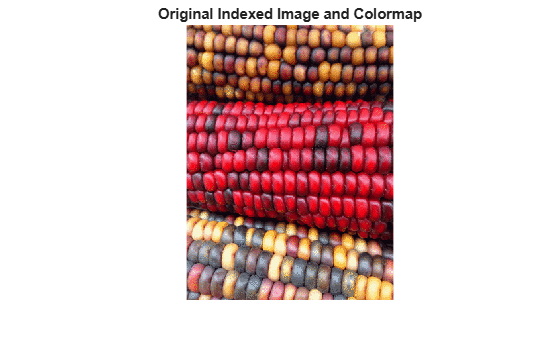```figure imshow(Y, newmap)```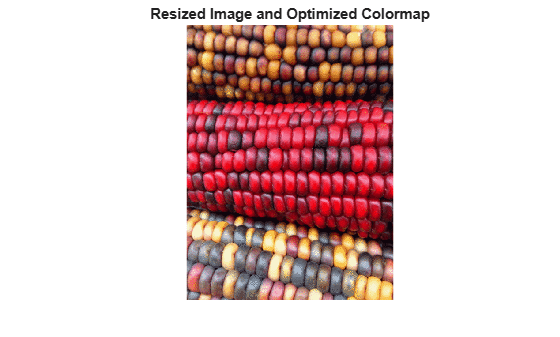## Input Arguments

collapse all

Image to be resized, specified as a real, nonsparse numeric array.

Data Types: `single` | `double` | `int8` | `int16` | `int32` | `uint8` | `uint16` | `uint32` | `logical`

Resize factor, specified as a real, numeric scalar.

Data Types: `single` | `double` | `int8` | `int16` | `int32` | `int64` | `uint8` | `uint16` | `uint32` | `uint64`

Row and column dimensions of output image, specified as a two-element numeric vector of positive values. Either `numrows` or `numcols` can be `NaN`, in which case `imresize` computes the number of rows or columns automatically to preserve the image aspect ratio.

Data Types: `single` | `double` | `int8` | `int16` | `int32` | `int64` | `uint8` | `uint16` | `uint32` | `uint64`

Indexed image to be resized, specified as a real, nonsparse numeric array.

Example: ```[X2, newmap] = imresize(X,map,0.75);```

Data Types: `double` | `uint8` | `uint16`

Colormap associated with indexed image, m-by-3 numeric array.

Data Types: `double`

Interpolation method, specified as a character vector or two-element cell array.

When `method` is a character vector, it identifies a particular method or named interpolation kernel, listed in the following table.

MethodDescription

`'nearest'`

Nearest-neighbor interpolation; the output pixel is assigned the value of the pixel that the point falls within. No other pixels are considered.

`'bilinear'`

Bilinear interpolation; the output pixel value is a weighted average of pixels in the nearest 2-by-2 neighborhood

`'bicubic'`

Bicubic interpolation; the output pixel value is a weighted average of pixels in the nearest 4-by-4 neighborhood

### Note

Bicubic interpolation can produce pixel values outside the original range.

Interpolation KernelDescription
`'box'`Box-shaped kernel
`'triangle'`Triangular kernel (equivalent to `'bilinear'`)
`'cubic'`Cubic kernel (equivalent to `'bicubic'`)
`'lanczos2'`Lanczos-2 kernel
`'lanczos3'`Lanczos-3 kernel

When `method` is a two-element cell array, it defines a custom interpolation kernel. The cell array has the form {f,w}, where f is a function handle for a custom interpolation kernel and w is the width of the custom kernel. f(x) must be zero outside the interval -w/2 <= x < w/2. The function handle f can be called with a scalar or a vector input. For user-specified interpolation kernels, the output image can have some values slightly outside the range of pixel values in the input image.

Data Types: `char` | `cell`

### Name-Value Pair Arguments

Specify optional comma-separated pairs of `Name,Value` arguments. `Name` is the argument name and `Value` is the corresponding value. `Name` must appear inside quotes. You can specify several name and value pair arguments in any order as `Name1,Value1,...,NameN,ValueN`.

Example: ```I2 = imresize(I,0.5,'Antialiasing',false);```

Perform antialiasing when shrinking an image, specified as the comma-separated pair consisting of `'Antialiasing'` and the logical Boolean value `true` or `false`. The default value depends on the interpolation method. If the method is nearest-neighbor (`'nearest'`), the default is `false`. For all other interpolation methods, the default is `true`.

Data Types: `logical`

Return optimized colormap, specified as the comma-separated pair consisting of `'Colormap'` and the character vector `'optimized'` or `'original'`. (Indexed images only). If set to `'original'`, the output colormap (`newmap`) is the same as the input colormap (`map`). If set to `'optimized'`, `imresize` returns a new optimized colormap.

Data Types: `char`

Perform color dithering, specified as the comma-separated pair consisting of `'Dither'` and the logical Boolean value `true` or `false`. (Indexed images only).

In dithering, you apply a form of noise to the image to randomize quantization error and prevent large-scale patterns.

Data Types: `logical`

Interpolation method, specified as the comma-separated pair consisting of `'Method'` and a character vector or two-element cell array. For details, see `method`.

Data Types: `char` | `cell`

Size of the output image, specified as the comma-separated pair consisting of `'OutputSize'` and a two-element vector of the form `[numrows numcols]`.

Data Types: `single` | `double` | `int8` | `int16` | `int32` | `int64` | `uint8` | `uint16` | `uint32` | `uint64`

Resize scale factor, specified as the comma-separated pair consisting of `'Scale'` and a positive numeric scalar or two-element vector of positive values.

Data Types: `single` | `double` | `int8` | `int16` | `int32` | `int64` | `uint8` | `uint16` | `uint32` | `uint64`

## Output Arguments

collapse all

Resized image, returned as a real, nonsparse numeric array, the same class as the input image.

Resized indexed image, returned as a real, nonsparse numeric array, the same class as the input image.

Optimized colormap, returned as an m-by-3 numeric array.

## Tips

• If the size of the output image is not an integer, `imresize` does not use the scale specified. `imresize` uses `ceil` when calculating the output image size.